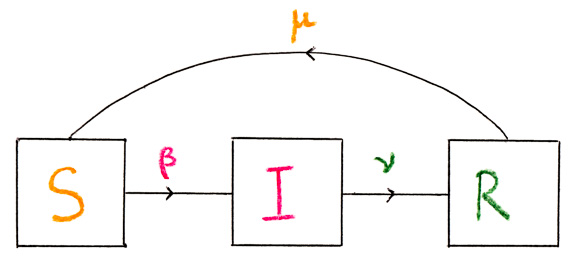Expérimentation Numérique Interactive

Accueil > Expériences en ligne > Dynamique des populations > A model of spread of infectious diseases

#A model of spread of infectious diseases

Rajouté le lundi 9 janvier 2017

Publié dans : "Differential Equations. An invitation through embedded visual interactive digital experiments" p 84. 2017

We study one of the simplest models used in epidemics. We divide a given population into three disjoint groups. The population of susceptible individuals is denoted by $S$, the infected population by $I$, and the recovered population by $R$. Of course, each of these is a function of time. We make the following assumptions:

• the total population is constant (no births or deaths), so that $\dot{P}=0$, where $P(t)=S(t)+I(t)+R(t)$. We denote by $P_0$ this constant;
• the rate of transmission of the disease is proportional to the number of encounters between susceptible and infected individuals. The easiest way to characterize this assumption mathematically is to put $\dot{S}=-\beta SI$ for some constant $\beta>0$ which represents the average number of contacts by an infected individual;
• The constants $\nu>0$ and $\mu>0$ represent the recovery rate and rate of loss of immunity, respectively.

We can schematically represent this model by the following diagram.We get the following equations: $$\begin{cases} \dot{S}= -\beta SI + \mu R \\ \dot{I}=\beta SI-\nu I\\ \dot{R}=\nu I -\mu R \end{cases}$$

At first glance, this is a three-dimensional system. In fact, it reduces to a two-dimensional one. Indeed, we can for instance eliminate $R$ since $$R=P_0-S-I.$$ Thus, if we determine $S(t)$ and $I(t)$, we then derive $R(t)$ for free. It results that the initial model boils down to the two-dimensional system $$\begin{cases} \dot{S}= -\beta SI + \mu (P_0-S-I) \\ \dot{I}=\beta SI-\nu I\\ \end{cases}$$ with four (positive) parameters $\beta,\mu,\nu$ and $P_0$.
We can check easily that there are at most two fixed points, one at $(P_0,0)$ and the other at $$(\bar{S},\bar{I})=\left( \frac{\nu}{\beta},\frac{\mu\big(P_0-\frac{\nu}{\beta}\big)}{\nu+\mu}\right).$$ The first fixed point clearly corresponds to no disease whatsoever in the population. The second fixed point only exists when $$P_0\geq \frac{\nu}{\beta}.$$ When $P_0=\nu/\beta$, we have a bifurcation as the two fixed points coalesce at $(P_0,0)$. The number $\nu/\beta$ is called the threshold level for the disease.
We can also find the $I$-nullclines which are given by $I=0$ and $S=\nu/\beta$, and the $S$-nullcline which is given by the graph of the function $$I(S)=\frac{\mu(P_0-S)}{\beta S+\mu}.$$

Notice that we are only interested by the triangular region of the plane $(S,I)$ given by $I,S\geq 0$ and $S+I\leq P_0$. Indeed, $S$ and $I$ represent population densities, and $P_0=S+I+R\geq S+I$. Therefore, the model is consistent if this region is invariant, in the sense that if we start in it, we stay there forever. Note that the $I$-axis is not invariant, while on the $S$-axis, solutions increase up to the fixed point $(P_0,0)$. One can check that the vector field along the boundary of this triangular region is either tangent or points inward.

Let's give more details. The Jacobian matrix at a point $(S,I)$ reads $$\begin{pmatrix} -\beta I-\mu & -\beta P_0-\mu \\ \beta I & \beta S-\nu \end{pmatrix}.$$ When $P_0>\nu/\beta$, the determinant of the Jacobian matrix at $(P_0,0)$ is equal to $\mu(\nu-\beta P_0)<0$. At the other fixed point, one checks that the determinant is equal to $\beta \bar{I}(\beta \bar{S}+\mu)>0$, and the trace is equal to $-\beta\bar{I}-\mu<0$. Hence, $(P_0,0)$ is a saddle, whereas $(\bar{S},\bar{I})$ is a sink.
When $P_0>\nu/\beta$, the only fixed point is $(P_0,0)$. The determinant of the Jacobian matrix at this point is $>0$, and the trace is $<0$. We thus have a sink.